ConicBundle

Minorant interface supporting Matrix classes; simple constructros for subgradients given by column vectors in form of a CH_Matrix_Classes::Matrix or a CH_Matrix_Classes::Sparsemat. More...

#include <MatrixCBSolver.hxx>

Inheritance diagram for ConicBundle::MatrixMinorant:## Public Member Functions

MatrixMinorant (CH_Matrix_Classes::Real offset, const CH_Matrix_Classes::Matrix &subg, PrimalData *primal=0, bool offset_at_origin=false)
initializaton by a Matrix column vector; if offset_at_origin==true, the offset gives the value at the origin (in contrast to the value at the current evaluation point)

MatrixMinorant (CH_Matrix_Classes::Real offset, const CH_Matrix_Classes::Sparsemat &subg, PrimalData *primal=0, bool offset_at_origin=false)
initializaton by a Sparsemat column vector; if offset_at_origin==true, the offset gives the value at the origin (in contrast to the value at the current evaluation point)

MatrixMinorant (const Minorant *mnrt, CH_Matrix_Classes::Real factor=1., bool with_primal=true)
generates a MatrixMinorat copy of a Minorant scaled by factor (deault =1.) and optionally without cloning the primal (if it exists)

Minorantclone_minorant (double factor=1., bool with_primal=true) const
produces a clone of itselfPublic Member Functions inherited from ConicBundle::Minorant
Minorant (double offset, const DVector &subg, PrimalData *primal=0, bool offset_at_origin=false)
Minorant constructor for a dense subgradient specified via a DVector. More...

Minorant (double offset, const DVector &subg_val, const IVector &subg_ind, PrimalData *primal=0, bool offset_at_origin=false)
Minorant constructor for a sparse subgradient where the nonzero values are specified by DVector and the corresponding indices by an IVector. More...

Minorant (bool offset_at_origin=true, double offset=0., int n_elementes=0, const double *coeffs=0, const int *indices=0, double scale_val=1., PrimalData *primal=0)
default initializes a zero minorant, see full explanation otherwise; NOTE: if the offset supplied by the minorant refers to the evaluation point (i.e., if it is the function value at the point of evaluation), set offset_at_origin = false ! More...

Minorant (const Minorant *mnrt, double factor=1., bool with_primal=false)
they main purpose of this constructor is to allow easy cloning for derived classes

virtual double offset () const
returns the current offset value

adds this value to the current offset value

virtual bool & offset_gives_value_at_origin ()
allows to specify/modify whether the offset refers to origin or to the point of evaluation at which this minorant was supplied

virtual bool offset_gives_value_at_origin () const
true if the offset refers to origin and false, if the offset refers to the value of the minorant in the point of the oracle evaluation at which this minorant was supplied

virtual double coeff (int i)
returns the value of the coefficient in coordinate i

virtual int add_coeff (int i, double value)
adds the value to the coefficient in coordinate i

virtual int add_coeffs (int n_elements, const double *values, double factor=1., int start_pos=0)
adds the n values (possibly multiplied by factor) to consecutive coefficients starting at start_pos (by default 0); this always converts the minroant into a dense vector first and adds then

virtual int add_coeffs (int n_elements, const double *values, const int *indices, double factor=1.)
adds the n values (possibly multiplied by factor) to the coefficients indicated by indices (if zero, this calls the other add_coeffs for the consecutive version)

virtual int sparsify (double tol=CB_minorant_zero_tolerance, double sparsity_ratio=CB_minorant_sparsity_ratio)
converts to sparse format with zeros of absolut value at most tol*(fabs(offset)+1) if the given ratio of elements is zero in relation to the maximum nonzero index

virtual int nonzeros ()
returns the number of nonzero coefficients

virtual int get_coeffs (int &n_elements, const double *&coeffs, const int *&indices) const
returns the list of all nonzero coefficients by returning pointers to the arrays and their common length n_elements More...

virtual double * get_dense_coeff_store (int n_elements)
If the returned pointer is not NULL it gives direct access to the array of current coefficient values with indices 0 up to n_elements-1;. More...

virtual int reassign_coeffs (int n_elements, const int *map_to_old_coeffs)
resorts (and deletes) coefficients so that afterwards it has n_elements and the new coeff(i) has the previous value of coeff(map_to_old_coeff(i))

virtual void set_primal (PrimalData *)
if the minorant is generated by PrimalData and this should be aggregated along, insert a heap object of it here (see PrimalData)

virtual PrimalDataget_primal ()
returns NULL if there is no primal data and otherwise a pointer to it

virtual const PrimalDataget_primal () const
returns NULL if there is no primal data and otherwise a pointer to it (const version)

virtual int aggregate (const Minorant &minorant, double factor=1.)
adds factor*minorant to this and does this also for the primal if it is availabe

virtual int number_aggregated () const
returns the number of minorants aggregated in this one, value 1 thus means not aggregated

virtual int scale_minorant (double scale_val)
mutliply offset and coefficients (and PrimalData, if given) by scale_val

virtual double norm_squared () const
return the squared Euclidean normProtected Member Functions inherited from ConicBundle::Minorant
Minorant (const Minorant &)
forbidden, blocked deliberately

Minorantoperator= (const Minorant &)
forbidden, blocked deliberatelyProtected Attributes inherited from ConicBundle::Minorant
MinorantData * data
implementation details are hidden on purpose

## Detailed Description

Minorant interface supporting Matrix classes; simple constructros for subgradients given by column vectors in form of a CH_Matrix_Classes::Matrix or a CH_Matrix_Classes::Sparsemat.

Suppose in the evaluation of your oracle at the current point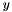you determine the subgradient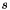for the subgradient inequality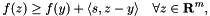then use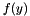for offset andfor subg in the constructors and keep the default value offset_at_origin == false.

If, on the other hand, your oracle implements a support function for some compact set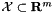like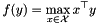then it is actually more efficient to return 0 for offset, a maximizing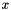in @ subg and to put offset_at_origin = true.

The documentation for this class was generated from the following file: